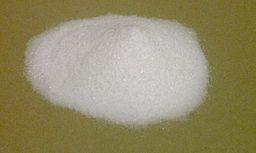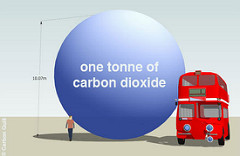Chemistry µGCSE:

Moles + Masses

10 quick questions - for GCSE and iGCSE

 10 minutes maximum! (can you do it in 5?) 1. In Chemistry, the unit for the amount of a substance is the … A. gram B. kilogram C. mole D. cubic decimeter (dm3) ? A B C D 2. The relative formula mass, Mr, of MgCl2 is …      A. 95 B. 46 C. 59.5 D. 95g ? A B C D 3. The molar mass of NaHCO3 is … A. 84 B. 84g C. 52 D. 52g? A B C D 4. Which of these is the correct formula for number of moles of a compound? A. number of moles = mass (in g) x relative formula mass (Mr) B. number of moles = mass (in g) ÷ relative formula mass (Mr) C. number of moles = mass (in kg) x relative formula mass (Mr) D. number of moles = mass (in kg) ÷ relative formula mass (Mr) ? A B C D 5. How many moles are there in 10g of NaOH ?    [Mrof NaOH = 40] A. 4 B. 400 C. 0.25 D. 0.00025 ? A B C D 6. What is the mass of 0.2 moles of CO2?  [Mr of CO2 = 44] A. 8.8g B. 220g C. 0.00454g D. 8.8kg ? A B C D Q7-8: A farmer bought 40kg of the fertilizer ammonium nitrate, NH4NO3 . 7. The relative formula mass of ammonium nitrate is…. A. 42 B. 80 C. 45 D. 23? A B C D 8. The amount, in moles, of ammonium nitrate fertilizer purchased is…. A. 0.5 B. 2.0 C. 500 D. 3200 ? A B C D 9. Carbon dioxide, CO2, is a greenhouse gas. How many moles in 1.00 tonne of carbon dioxide? [1 tonne = 1,000,000g] A. 0.0227 B. 22.7 C. 22 727 D. 44? A B C D 10. Which of the following contains the greatest number of atoms? A. 2 moles of carbon, C B. 36g of carbon, C C. 0.5 moles of magnesium, Mg D. 48g of magnesium, Mg ? A B C D